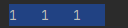# 一、基本使用方法

## 使用3步骤

### 1.不管三七二十一，先把基本内容写上

``print()``

### 2.然后在里面开始写想要输出的内容并使用 “ + ” 加号链接

``name_E = "Yorlen"  # 变量及字符串的使用age = 23 # 变量及整形的使用hobby_E = ["swimming", "singing", "reading", "travel"]print("My name is" + name_E + ", I'm " + age + " years old! My hobby is " + hobby_E + ".")``

``name_C = "小张"age = 23 # 变量及整形的使用hobby_C = ["游泳", "唱歌", "读书", "旅游"]print("我的名字是" + name_C + "我今年" + age + "岁了，我的爱好是" + hobby_C + "。")``

### 3.调整输出内容的数据类型，如 类型转换，列表/字典等特殊数据类型的特殊处理

``# 变量及字符串的使用name_E = "Yorlen"# 变量及整形的使用age = 23hobby_E = ["swimming", "singing", "reading", "travel"]print("My name is" + name_E + ", I'm " + str(age) + " years old! My hobby is " + "、 ".join(hobby_E) + ".")``

结果

``My name isYorlen, I'm 23 years old! My hobby is swimming、 singing、 reading、 travel.``

``name_C = "小张"age = 23hobby_C = ["游泳", "唱歌", "读书", "旅游"]print("我的名字是" + name_C + "我今年" + str(age) + "岁了，我的爱好是" + "、 ".join(hobby_C) + "。")``

结果

``我的名字是小张我今年23岁了，我的爱好是游泳、 唱歌、 读书、 旅游。``

# 二、内置函数说明

``def print(self, *args, sep=' ', end='\n', file=None): # known special case of print    """    print(value, ..., sep=' ', end='\n', file=sys.stdout, flush=False)        Prints the values to a stream, or to sys.stdout by default.    Optional keyword arguments:    file:  a file-like object (stream); defaults to the current sys.stdout.    sep:   string inserted between values, default a space.    end:   string appended after the last value, default a newline.    flush: whether to forcibly flush the stream.    """    pass``

sep = separate  分开，分离，间隔

end  末尾，结束

file  文件

## 1.sep 参数的使用

print()方法，除了使用  “+”加号  连接输出内容之外，还可以使用  “,”逗号  连接输出内容。

``print(23, "Yorlen", "kbhit")print(str(23) + "Yorlen" + "kbhit")``

``23 Yorlen kbhit23Yorlenkbhit``

观察分别使用  “,”逗号  和  “+”加号  连接输出的区别

``print(23, "Yorlen", "kbhit", sep="")print(str(23) + "Yorlen" + "kbhit")``

结果

``23Yorlenkbhit23Yorlenkbhit``

我们尝试将sep参数改变成其他内容看看什么效果

``print(23, "Yorlen", "kbhit", sep="***")``

结果

``23***Yorlen***kbhit``

可以看到sep的作用就是当我们使用  “,”逗号  连接输出内容时，输出内容之间可以使用sep参数设置间隔内容。

## 2.end 参数的使用

end参数的使用与sep参数的使用方法类似，只不过end会在每一个print的结尾改变打印内容

### 不修改end

``print(1)print(1)print(1)``

``111 ``

### 修改end参数内容

``print(1, end="\t")print(1, end="\t")print(1, end="\t")``

``1	1	1	``

看下图，最后一个1后面还是有4个空格的### 使用不同的end内容

``print(1, end="\t")print(1, end="\t")print(1, end="****")``

结果

``1	1	1****``

## 3. file 参数的使用

``f = open(r"123.txt","w")print(123,file=f)``

在该python文件的同级目录中，可以找到一个名叫”123.txt”的文件，打开查看，可以看到里面存储内容为   222

# 三、print源码

``def print(*args, **kargs):    sep = kargs.get('sep', " ")    end = kargs.get('end', '\n')    file = kargs.get('file', sys.stdout)    output = ""    first = True    for arg in args:        output += ("" if first else sep) + str(arg)        first = False    file.write(output + end)``